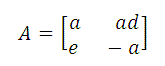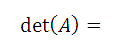# Free Compass Math Test Practice Questions Solutions with Explanations - Sample 4

 Solutions with detailed explanations to compass math test practice questions in sample 4. If f(x) = 2x - 3, g(x) = -2x3 + 2 and h(x) = 3x, then h(2) + f(g(-1)) = Solution We first calculate h(2) h(2) = 3(2) = 6 We next calculate g(-1) g(-1) = -2(-1)3 + 2 = -2(-1) + 2 = 4 We next calculate f(g(-1)) f(g(-1)) = f(4) = 2(4) - 3 = 8 - 3 = 5 Finally h(2) + f(g(-1)) = 6 + 5 = 11 If 3x2 / 34x = 1/81, then x = Solution We first rewrite the two expressions in the given equation to the same base 3x2 / 34x = 3x2-4x and 1/81 = 1 / 34 = 3-4 We now rewrite the equation as follows 3x2-4x = 3-4 Which leads to the following algebraic equation x2 - 4x = -4 x2-4x + 4 = 0 factor and solve (x - 2)2 = 0 x = 2 What are the solutions of x(x - 2) = 5? Solution Expand and write in standard form x2 - 2x - 5 = 0 Use quadratic formulas a x = [ -(-2) + or - sqrt( (-2)2 - 4(1)(-5)) ] / 2 = [ 2 + or - sqrt(24) ] / 2 = [ 2 + or - 2 sqrt(6) ] / 2 two solutions: 1 + sqrt(6) and 1 - sqrt(6) The formula for the nth term, an, of an arirhmetic progression is given by an = a1 + (n - 1)d, where a1 represents the first term of the progession and d represents its common difference. What is the value of the 20th term of the arithmetic progression 4, 7, 10,...? Solution The common difference of the given progression is 7 - 4 = 3 (or 10 - 7 = 3) The first term is a1 = 4 The value of the 20th term is given by a20 = a1 + (n - 1)d = 4 + (20 - 1)3 = 4 + 3 × 19 = 4 + 57 = 61 In a a 16-by-12 rectangle, what is the perimeter of the triangle formed by two sides of the rectangle and the diagonal? Solution Let x be the length of the diagonal and use Pythagora's theorem to find it. x2 = 162 + 122 = 256 + 144 = 400 x = sqrt(400) = 20 The three sides of the triangle are the length and width of the rectangle and the diagonal. Perimeter = 16 + 12 + 20 = 48 If sqrt(-1) = i, then (-2 + 2 i)5 = Solution We first write the complex number z = (-2 + 2i) in exponential form. z = (-2 + 2i) = sqrt((-2)2 + (22)) e[ i arg(2/-2) ] = 2 sqrt(2) e[ i arg(2/-2) ] = 2 sqrt(2) e[ 3 Pi/4 i ] We now apply De Moivre theorem to find (-2 + 2 i)5 (-2 + 2 i)5 = z 5 = (2 sqrt(2))5 e[ 5 · 3 Pi/4 i ] = 128 sqrt(2) · e[ 15 Pi/4 i ] = 128 *sqrt(2)* [ sin(15 Pi/4) + i cos(15 Pi/4) ] = 128 sqrt(2) [1/sqrt(2) - i * 1/sqrt(2) ] = 128 - 128 i For all values of x > 0, log[( x2 cube root(7) ] = Solution We first the log formula log (A*B) = log A + log B to write that log[( x2 cube root(7) ] = log( x2) + log(cube root(7)) We now use the log formula log (An) = n log A to simplify the above = 2 log x + log (7(1/3)) = 2 log x + (1/3) log 7 Which of these interval represents all the real values that are the range of y = 1 / (4 - x2) Solution y = 1 / (4 - x2) is a even function since y(-x) = 1 / (4 - (-x)2) = 1 / (4 - x2) Because it is an even function and therefore its graph is symmetric with respect to the y axis, we shall study its graph for x ? 0. Since the denominator of this rational function is zero at x = + or -2, it has 2 vertical asymptotes: x = 2 and x = - 2. It also has a horizontal asymptote given by y = 0 because the degree of the denominator is greater than the degree of the numerator. For 0 ? x < 2, 4 - x2 is positive and therefore 1 / (4 - x2) is also positive. For x > 2 , 4 - x2 is negative and therefore 1 / (4 - x2) is also negative. Also for x = 0, y = 1/4 Putting all the above information together to graph the given function, we end up with the graph below.. The range of the given function is given by the interval. (-infinity , 0) U [1/4 , +infinity) Which of these intervals represents all values of x that makes sqrt(x2 + 2x) a real number? Solution sqrt(x2 + 2x) is real if . x2 + 2x ? 0 or x(x + 2) ? 0 x(x + 2) changes sign at the values of x that make x(x + 2) = 0 which are x = 0 and x = - 2. Hence 3 intervals to study the sign of x(x + 2) interval 1: (- infinity , -2] , x(x + 2) ? 0 interval 2: [-2 , 0] , x(x + 2) ? 0 interval 3: [0 , +infinity) , x(x + 2) ? 0 sqrt(x2 + 2x) is real for x in the interval (-infinity , -2] U [0 , +infinity) log 32 + log 16 = Solution Note that 32 = 25 and 16 = 24 Hence log 32 + log 16 = log 25 + log 24 = 5 log 2 + 4 log 2 = 9 log 2 If 9(x + 1) = 3 * 9y, then Solution Let us rewrite the two sides of the equation to the same base 9(x + 1) = (32)(x + 1) = 32(x + 1) 3 * 9y = 31 * (32)y = 31 + 2y We now rewrite the given equation as follows 32(x + 1) = 31 + 2y Which leads to 2(x + 1) = 1 + 2y Solve for x 2x + 2 = 1 + 2y 2x = 2y - 1 If A is a matrix given by, thenSolution The determinant of a 2 by 2 matrix of the formis given by AD - BC Apply the above to the given matrix to obtain the determinant as follows a(-a) - (ad)(e) = -a2 - aed = -a(a + ed) If cos(80o) = a and cos(60o)cos(20o) = b, then sin(60o)sin(20o) = Solution Write cos(80o) as follows cos(80o) = cos(60o + 20o) Expand = cos(60o)cos(20o) - sin(60o) sin(20o) Substitute cos(80o) by a and cos(60o)cos(20o) by b a = b - sin(60o) sin(20o) Hence sin(60o) sin(20o) = b - a Which pair of functions have the same graph? A) sin(x) and cos(x + 3 Pi/2) B) sin(x) and cos(x + Pi/2) C) sin(x) and cos(x + Pi) D) sin(x) and cos(x - 3 Pi/2) E) sin(x) and cos(x + 5 Pi/2) Solution Let us expand cos(x + 3 Pi/2) and simplify cos(x + 3 Pi/2) = cos(x) cos(3 Pi/2) - sin(x) sin(3 Pi/2) = cos(x) 0 - sin(x)(-1) = sin(x) Since cos(x + 3 Pi/2) simplify to sin(x), the two functions have the same graph. NOTE: As an exercise, expand the remaining functions: cos(x + Pi/2) , cos(x + Pi), cos(x - 3 Pi/2) and cos(x + 5 Pi/2) and show that none is equal to sin(x). Which of these functions have the highest period? A) cos(x + 3 Pi/2) B) sin(2x - Pi) C) cos(0.2x) D) 10 sin(x) E) cos(200x) Solution The period of a function of the form y = a sin(bx + c) or y = a cos(bx + c) is given by 2 Pi / |b| Let us now find the periods of the given functions A) cos(x + 3 Pi/2) : Period = 2 Pi / |1| = 2 Pi B) sin(2x - Pi) : Period = 2 Pi / |2| = Pi C) cos(0.2x) : Period = 2 Pi / |0.2| = 10 Pi D) 10 sin(x) : Period = 2 Pi / |1| = 2 Pi E) cos(200x) : Period = 2 Pi / |200| = Pi / 100 = 0.01 Pi The function with the highest period is cos(0.2x) What is the measure of x, 0 < x < 5 Pi / 2, if |- sin(x) + 1| = 2 Solution If |- sin(x) + 1| = 2 , then -sin(x) + 1 = 2 or -sin(x) + 1 = -2 sin(x) = - 1 or sin(x) = 3 The equation sin(x) = 3 has no real solutions. However the solution for the equation sin(x) = - 1 such that 0 < x < 5 Pi / 2 is x = 3 Pi/2 If Pi < x < 2 Pi and cos(x) = - 1 / 2, then x = Solution Start with cos(Pi / 3) = 1/2 For Pi < x < 2 Pi and cos(x) = - 1/2, x is in quadrant III. Hence x = Pi + Pi/3 = 4 Pi/3 [ cos(t) / cot(t) ] csc2(t) = Solution Use the identities csc(t) = 1 / sin(t) and tan(t) = 1 / cot(t) to write [ cos(t) / cot(t) ] csc2(t) = cos(t) tan(t) / sin2(t) = cos(t) [ sin(t) / cos(t)] / sin2(t) simplify = 1 / sin(t)= csc(t) If f(u) = 2 cos(u) + 5 and g(v) = 0.5 sin(2v), what is f(g(Pi / 2)) Solution We first calculate g(Pi/2) g(Pi/2) = 0.5 sin(2 Pi/2) = 0 f(g(Pi/2)) is calculated as follows f(g(Pi / 2)) = f(0) = 2 cos(0) + 5 = 2 * 1 + 5 = 7 If Pi < x < 2 Pi and tan(x) = 1/4, then sin(x) = Solution Use the definition of tan(x) = b / a where point (a,b) is on the terminal side of angle x to write tan(x) = 1/4 = b / a Since x is in quadrant III, we can write b = - 1 and a = - 4 r distance from (0,0) to (a,b) is given by r = sqrt(12 + 42) = sqrt(17) We now use sin(x) = b / r sin(x) = -1 / sqrt(17) More ACT, SAT and Compass practice